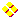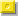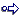HOME plateWON | World!OfNumbersPalindromic Products ofIntegers and their ReversalsSum of First NumbertypesSequence ProductsPythagorean TriplesPalindromes in other BasesPalindromes in ConcatenationsVarious Palindromic Sums

Introduction

Palindromic numbers are numbers which read the same fromleft to right (forwards) as from the right to left (backwards)Here are a few random examples : 505, 137731, 100246642001

Palindromic Products of Non palindromic Integers and their Reversals are defined and calculated by this extraordinary intricate
and excruciatingly complex formula. So, this line is for experts onlybase  x  esab

Palindromes and Reversals

Here is a gem from Carlos Rivera's puzzle website ( Source Puzzle 52 ).

Palindromic sum of two consecutive primes 32213 & 32233 equal to the sum of their reversibles

32213 + 32233 =  64446  = 33223 + 31223

A 3-digit number trick.

Everybody must have heard of the following number trick that involves 'reverse & add'-ing
the digits of a random 3-digit number. If not here your chance to catch up.

I'm grateful to Mitch Beck for making me aware of this property of 1089, a truly funny number.

Take any three integers from zero to nine, then subtract its reversal.
Then, if the difference is positive, add its reversal.
If the difference is negative, then subtract its reversal.
NO MATTER WHAT 3-DIGIT INTEGERS YOU BEGIN WITH, THE FINAL ANSWER IS ALWAYS 1089 !

```            856        159        872
- 658      - 951      - 278
------     ------     ------
198      - 792        594
+ 891      - 297      + 495
------     ------     ------
1089      -1089       1089
```

"Curious and Interesting Numbers", by David Wells, page 163, about 1089 :
If a 3-digit number is reversed and the result subtracted,
623 - 326 = 297 and 297 + 792 = 1089.

"Recreations in the Theory of Numbers", by Albert H. Beiler, page 63, says :
A common trick with an almost infinite number of variations is to
have someone write a three-digit number, then write the number
with the digits in reverse order, subtract the smaller from the larger,
reverse the digits again and add this time; you will then be able to
number. The answer is 1089. Suppose any number, say 173, were
taken. Subtract from 371, leaving 198, reverse, giving 891, and thev
sum of the last two numbers is 1089.

On the following page 64 Beiler gives a mathematical proof why this operation always works.
It might be worthwhile to order this book as it is almost a bible for number theory enthousiasts.

[ September 10, 2002 ]
Sathiya Subramanian (email) from Redwood City, California
struggled with what he thought is a counterexample of the above 3-digit number trick.

758
– 857
--------
– 99
– 99
--------
– 189 ??
The trick here is to keep using three digits even if a leading zero
appears in the result ! Sathiya's example thus becomes ¬
758
– 857
--------
– 099
– 990
--------
– 1089 !!
A very sharp observation nevertheless. Thanks!

PS. 1089 is also known for another conjecture.
When an integer and its reversal are unequal, their product is never a square except when both are squares.
1089 = 332 and 9801 = 992
We see that 1089 x 9801 = 10673289 which is 32672.

Some observations about Palindromic Products of Integers & their Reversals.

The basenumbers are made up of a combination of only three digits namely 0, 1 and 2 !
Although it is not the case, one could mistakenly believe that we are dealing with numbers in base 3 !!
Is someone prepared to find out why that is ? A mathematical proof will be much appreciated.

[ August 9, 2000 ]
Henry Bottomley (email) cannot quite give a proof yet, but his explanation is no less interesting.

```If the sum of the squares of the digits of n is less than 10 and n is not
divisible by 10, then the product of n and the reversal of n is a palindrome.
[Indeed extending this to any base k: if the sum of the squares of the base k
digits of n is less than k and n is not divisible by k, then the product of n
and the base k reversal of n is a base k palindrome.]

The reason for this is:
(i) any (perhaps partial) sum of pairwise products of digits of n is less than
or equal to the sum of the square of the digits of n, which in turn is less
than 10 [or k];
(ii) therefore there are no carries when calculating the digits of the product
of n and its reversal;
(iii) since multiplication and addition are commutative, this means that each
digit of the product is equal to the digit an equal distance the other side
of the middle of the product;
(iv) and since n is not a multiple of 10 [or k] there are no difficulties with
final zeros in the product.

To take your example, the digits of base*esab are (before any carries):
b*e; b*s+a*e; b*a+a*s+s*e; b*b+a*a+s*s+e*e; a*b+s*a+e*s; s*b+e*a; e*b
All of these are less or equal to b*b+a*a+s*s+e*e which is less than 10
[or k], so there are no carries.
So this appears to be a palindrome.
Since e is not zero (and neither is b), e*b is not zero, and so indeed this
is a palindrome.

If the condition is not met, palindromes are (probably) impossible, because
of the carries.

So the possibilities for digits are:
```
• 3;
• two 2s, with one or zero 1s, and as many 0s as required (but not as a final digit);
• one 2, with from zero through to five 1s, and as many 0s as required (but not as a final digit);
• from two through to nine 1s, and as many 0s as required (but not as a final digit);
• 1;
• 0 (as a special case).

Explorations into the reversal world

The table lists all the palindromes only upto length 9. Larger ones are very easy to find.
Per given length their total number grows exponentially.

```     Length of      Total genuine
basenumber     n x n_reversed
1               0
2               1
3               3
4              10
5              19
6              44
7               ?
```
That doesn't mean that we can't find some larger palindromes with beautiful patterns or special properties.
On the contrary. What about repunital palindromes except for the middle digit :
1011 x 1101 = 1113111
1010011 x 1100101 = 1111114111111
I'd like to name these palindromes wingnumbers, for obvious reason.
Here is another one from More Palindromic Products of Integer Sequences :
1051 x 1061 = 1115111
This same palindromic wingnumber has also another strange property (See Sloane's A032735).
It cannot be prefixed or followed by any digit from (1,2,3,4,5,6,7,8,9) to form a prime.
211151117, 511151115, 911151116, etc. are all composite !

And this wingnumber comes from the Nine Digits Page :
18 + 25 + 39 + 42 + 53 + 61 + 77 + 86 + 94 = 1112111

Fascinating digit-swapping occurs many times with e.g. these two reversals :

```    1 0 2 1 x 1 2 0 1 = 1 2 2 6 2 2 1
X     X         X         X
1 0 1 2 x 2 1 0 1 = 2 1 2 6 2 1 2
```
Here is a finite pattern starting with the very first one of the table :
2 x 2 = 4
12 x 21 = 252
112 x 211 = 23632
1112 x 2111 = 2347432
11112 x 21111 = 234585432
111112 x 211111 = 23456965432
Infinite patterns are abundant here. But this one has something special ! Read on.

1121 x 1211 = 1357531
1010201 x 1020101 = 1030507050301
1001002001 x 1002001001 = 1003005007005003001
1000100020001 x 1000200010001 = 1000300050007000500030001

And the following phenomenon totally surprised me !
All the first terms of the above pattern when added together with the square of their previous (less one) numbers
( see Quasi-Over-Squares ) also produce palindromes :
11202 + 1121 = 1255521
10102002 + 1010201 = 1020505050201
10010020002 + 1001002001 = 1002005005005002001
10001000200002 + 1000100020001 = 1000200050005000500020001

David Wilson found out an interesting palindrome-related fact
Article emailed at [ Sun, 08 Mar 1998 ]

```The number 1089 (the square of palindrome 33) is interesting
because it reverses when multiplied by 9: 9*1089 = 9801.
Well, it turns out that multiplication by 9 reverses a positive
integer if and only if that integer is of the form 99*p, where
p is a palindrome consisting of only the digits 0 and 1, in
which every run of 0's or 1's is at least two digits long.

For instance, the palindrome 11000111100011 fits the bill for p.
Then 99*p = 1089010998901089, and we see that

9*1089010998901089 = 9801098990109801.

Similarly, the set of numbers which reverses when multiplied
by 4 are those of the form 198*p, where p is of the same
above-described palindromic form.

Finally, it is obvious that the palindromes themselves are
reversed when multiplied by 1.  This exhausts the posibilities;
in base 10, the only multipliers that can reverse a positive
integer are 1, 4, or 9.

There is some evidence that similar types of things happen
in other bases.  For instance, in base 5, a number of the
form 13*p, where p is any palindrome consisting of 0's and 1's
is reversed when multiplied by 2.
```

More Sources Revealed

Eric Weisstein added an interesting article to his Math Encyclopedia about Reversals which are integral multiples of themselves.

2178 x 4 = 8712
219...978 x 4 = 879...912

I cannot withhold the following equation althought the numbers are not palindromic :
[ Source "Curious and Interesting Numbers" by David Wells, page 158 ]

651 x 156 = 372 x 273

```

```

The Table

Index NrInfo BasenumberLength
Palindromic Product of Integers & their ReversalsLength

Integer[Normal]  x  Integer[Reversed]
33 20.012 x 21.0025
420.292.0249
32 12.002 x 20.0215
240.292.0429
31 11.121 x 12.1115
134.686.4319
30 11.112 x 21.1115
234.585.4329
29 11.102 x 20.1115
223.272.3229
28 11.021 x 12.0115
132.373.2319
27 11.012 x 21.0115
231.373.1329
26 11.002 x 20.0115
220.161.0229
25 10.211 x 11.2015
114.373.4119
24 10.202 x 20.2015
206.090.6029
23 10.121 x 12.1015
122.474.2219
22 10.112 x 21.1015
213.373.3129
21 10.111 x 11.1015
112.242.2119
20 10.102 x 20.1015
203.060.3029
19 10.022 x 22.0015
220.494.0229
18 10.021 x 12.0015
120.262.0219
17 10.012 x 21.0015
210.262.0129
16 10.011 x 11.0015
110.131.0119
15 10.002 x 20.0015
200.050.0029

14 2.012 x 2.1024
4.229.2247
13 1.202 x 2.0214
2.429.2427
12 1.121 x 1.2114
1.357.5317
11 1.112 x 2.1114
2.347.4327
10 1.102 x 2.0114
2.216.1227
9 1.022 x 2.2014
2.249.4227
8 1.021 x 1.2014
1.226.2217
7 1.012 x 2.1014
2.126.2127
6 1.011 x 1.1014
1.113.1117
5 1.002 x 2.0014
2.005.0027

4 122 x 2213
26.9625
3 112 x 2113
23.6325
2 102 x 2013
20.5025

1 12 x 212
2523

Contributions

David W. Wilson (email) found out an interesting palindrome-related fact - go to topic

Henry Bottomley (email) gives an explanation why the basenumbers are composed
only of digits 0, 1 and 2 - go to topic

```

```

[TOP OF PAGE]

Patrick De Geest - Belgium- Short Bio - Some Pictures